RS Aggarwal Class 9 Solutions Chapter 9 - Quadrilaterals And Parallelograms Ex 9A (9.1)

RS Aggarwal Class 9 Chapter 9 - Quadrilaterals And Parallelograms Ex 9A (9.1) Solutions Free PDF

Q.1: In the adjoining figure, ABCD is a parallelogram in which ∠A = 72°. Calculate ∠B, ∠C and ∠D.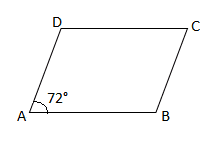Sol:

ABCD is a parallelogram and ∠ A = 72°

We know that opposite angles of a parallelogram are equal

∴ ∠A = ∠C and ∠B = ∠D

∴ ∠C = 72°

∠A and ∠B are adjacent angles

i.e., ∠A + ∠B = 180°

$\Rightarrow$ ∠B = 180° – ∠A

$\Rightarrow$∠B = 180° – 72° = 108°

∠B = ∠D =108°

Hence, ∠B = ∠D = 108° and ∠C = 72°

Q.2: In the adjoining figure, ABCD is a parallelogram in which ∠ DAB = 80° and ∠ DBC = 60°. Calculate ∠ CDB and ∠ ADB.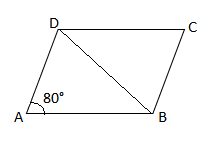Sol:

Given: ABCD is a parallelogram and ∠ DAB = 80° and ∠ DBC = 60°

To find: Measure of ∠CDB and ∠ ADB

In parallelogram ABCD, AD $\parallel$ BC

∴ ∠ DBC = ∠ ADB = 60° (Alternate interior angles) . . . . . . . (i)

∴∠ ADC = 180° – ∠ DAB

∠ ADC = 180° – 80° = 100°

∠ ADB + ∠ CDB = 100° . . . . . . . (ii)

From (i) and (ii), we get:

60° + ∠ CDB = 100°

∠ CDB = 100° – 60° = 40°

Hence, ∠ CDB = 40° and ∠ ADB = 60°

Q.3: In the adjoining figure, ABCD is a parallelogram in which ∠ A = 60°. If the bisectors of ∠ A and ∠ B meet DC at P, prove that (i) ∠ APB = 90°, (ii) AD = DP and PB = PC = BC, (iii) DC = 2AD.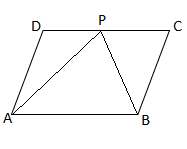Sol:

ABCD is a parallelogram

∴ ∠ A = ∠ C and ∠ B = ∠ D (Opposite angles)

And ∠ A + ∠ B = 180° (Adjacent angles are supplementary)

∴ ∠ B = 180° – ∠ A

180° – 60° = 120° (Since, ∠ A = 60°)

∴ ∠ A = ∠ C = 60° and ∠ B = ∠ D = 120°

(i) In Δ$\Delta$ APB, ∠ PAB = 602$\frac{60^{\circ}}{2}$ = 30° and ∠ PBA = 1202$\frac{120^{\circ}}{2}$ = 60°

∴ ∠ APB = 180°- (30° + 60°) = 90°

(ii) In Δ$\Delta$ ADP, ∠PAD = 30° and ∠ADP = 120°

∴ ∠APB = 180° – (30° + 120°) = 30°

Thus, ∠PAD = ∠APB = 30°

Hence, Δ$\Delta$ADP is an isosceles triangle and AD = DP.

In triangle PBC, ∠PBC = 60°. ∠BPC = 180° – (90° – 30°) = 60° (Opposite angle of ∠A)

∠PBC = ∠BPC = ∠BCP

Hence, Δ$\Delta$ PBC is an equilateral triangle and, therefore, PB = PC = BC

(iii) DC = DP + PC

From (ii), we have:

Hence, Δ$\Delta$PBC is an equilateral triangle and, therefore, PB = PC = BC.

DC = DP + PC

From (ii) we have:

Q.4: In the adjoining figure, ABCD is a parallelogram in which ∠BAO = 35°, ∠DAO =

40° and ∠COD = 105°.

Calculate (i) ∠ABO, (ii) ∠ODC, (iii) ∠ACB, (iv) ∠CBD

Sol:

ABCD is a parallelogram.

AB || DC and BC || AD

(i) In Δ$\Delta$AOB, ∠BAO = 35°, ∠A0B = ∠COD = 105° (Vertically opposite angels)

∠ABO = 180° – (35° + 105°) = 40°

(ii) ∠ODC and ∠ABO are alternate interior angles

∠ODC = ∠ABO = 40°

(iii) ∠ACB = ∠CAD = 40° (Alternate interior angles)

(iv) ∠CBD = ∠ABC – ∠ABD

∠ABC = 180° – 75° = 105°

∠LCBD = 105° – ∠ABD

∠CBD = 105° – 40° = 65° (Adjacent angles are supplementary) and cABD = cABO

Q.5: In a parallelogram ABCD, if ∠A = (2x+25) ° and ∠B = (3x-5) °, find the value of X and the measure of each angle of the parallelogram.

Sol:

ABCD is a parallelogram

i.e., ∠A = ∠C and ∠B = ∠D (Opposite angles)

Also, ∠A + ∠B = 180° (Adjacent angles are supplementary)

(2x + 25) ° + (3x — 5) ° = 180

5x +20 = 180

5x =160

x = 32°

∠A= 2 x 32 + 25 = 89° and ∠B = 3 x 32 — 5 = 91°

Hence, x = 32°, ∠A = ∠C = 89° and ∠B = ∠D = 91°

Q.6: If an angle of a parallelogram is four-fifths of its adjacent angle, find the angles of the parallelogram.

Sol:

Let, ABCD be a parallelogram

∠A = ∠C and ∠B = ∠D (Opposite angles)

Let ∠A = x° and ∠B = (4x/5)°

Now, ∠A + ∠B = 180° (Adjacent angles are supplementary)

x + (4x/5) = 180°   => 9x/5 = 180° i.e. x = 100°

Now, ∠A = 100° and ∠B = (4/5) x 100° = 80°

Hence, ∠A = ∠C = 100°; ∠B = ∠D = 80°

Q.7: Find the measure of each angle of a parallelogram, if one of its angles is 30° less than twice the smallest angle.

Sol:

Let ABCD be a parallelogram.

∠A = ∠C and ∠B = ∠D (Opposite angles)

Let ∠A. be the smallest angle whose measure is x°.

∠B = (2x – 30)°

Now, ∠A. + ∠B = 180° (Adjacent angles are supplementary)

x + 2x – 30° = 180°

3x = 210°

x = 70°

∠B = 2 x 70° – 30° = 110°

Hence, ∠A. = ∠C = 70°; ∠B = ∠D = 110°

Q.8: ABCD is a parallelogram in which AB = 9.5cm and its perimeter is 30cm. Find the length of each side of a parallelogram.

Sol:

ABCD is a parallelogram

The Opposite sides of a parallelogram are parallel and equal

∴ AB = DC = 9.5 cm

Let, BC = AD = x

∴ Perimeter of ABCD = AB + BC + CD + DA = 30cm

$\Rightarrow$ 9.5 + x + 9.5 + x = 30

$\Rightarrow$ 19 + 2x = 30

$\Rightarrow$ 2x = 11

$\Rightarrow$ x = 5.5 cm

Hence, AB = DC = 9.5 cm and BC = DA = 5.5 cm

Q.9: In each of the figures given below, ABCD is a rhombus. Find the value of X and Y in each case.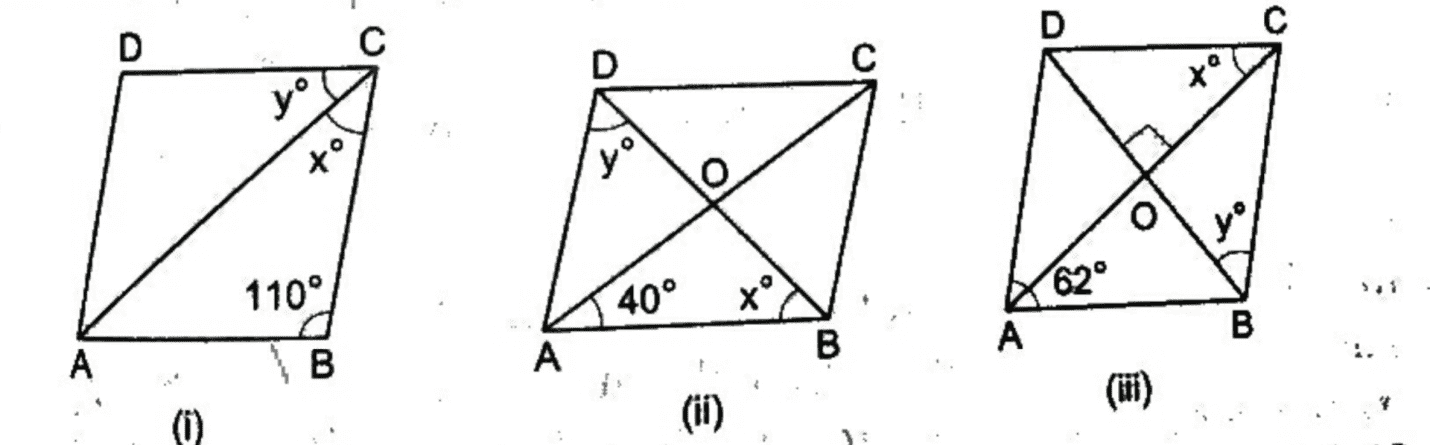Sol:

ABCD is a rhombus and a rhombus is also a parallelogram. A rhombus has four equal sides.

(i) In ΔABC$\Delta ABC$, ∠ BAC = ∠ BCA = 12(180110)$\frac{1}{2}(180^{\circ}-110^{\circ})$ = 35°

i.e., x = 35°

Now, ∠B + ∠ C = 180°(Adjacent angles are supplementary)

But, ∠C = x + y = 70°

$\Rightarrow$ y = 70° – x

$\Rightarrow$ y = 70° – 35° = 35°

Hence, x = 35°and y = 35°

(ii) The diagonals of a rhombus are perpendicular bisector of each other.

So, in ΔAOB$\Delta AOB$, ∠OAB = 40°, ∠ AOB = 90 °and ∠ ABO = 180°- (40° + 90°) = 50 °

∴ x = 50 °

In ΔABD$\Delta ABD$, AB = AD

So, ∠ ABD = ∠ ADB = 50°

Hence, x = 50°and y = 50°

(iii) ∠ BAC = ∠ DCA (Alternate interior angles)

i.e., x = 62°

In ΔBOC$\Delta BOC$, ∠ BCO = 62° [In ΔABC$\Delta ABC$, AB = BC, so ∠BAC = ∠ ACB]

Also, ∠BOC = 90°

∴ ∠ OBC = 180° – (90° + 62°) = 28°

Hence, x = 62°and y = 28°

Q.10: The lengths of the diagonals of a rhombus are 24cm and 18cm respectively. Find the length of each side of the rhombus.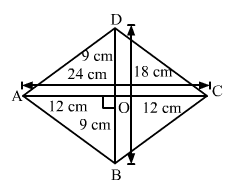Sol:

Let, ABCD be a rhombus

∴ AB = BC = CD = DA

Here, AC and BD are the diagonals of ABCD, where AC = 24 cm and BD = 18 cm

Let, the diagonals intersect each other at O

We know that the diagonals of a rhombus are perpendicular bisectors of each other

ΔAOB$\Delta AOB$  is a right angle triangle in which OA = AC / 2 = 24 / 2 = 12 cm

And OB = BD / 2 = 18 / 2 = 9 cm.

Now, AB2 = OA2 + OB2 (Pythagoras theorem)

$\Rightarrow$ AB2 = (12)2 + (9)2

$\Rightarrow$ AB2 =144 + 81 = 225

$\Rightarrow$AB = 15 cm

Hence, the side of the rhombus is 15 cm

Q.11: Each side of a rhombus is 10cm long and one of its diagonals measures 16cm.Find the length of the other diagonal and hence find the area of the rhombus.

Sol: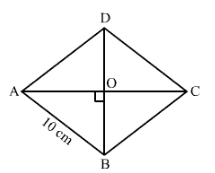Let, ABCD be a rhombus

AB = BC = CD = DA = 10cm

Let, AC and BD be the diagonals of ABCD. Let AC = x and BD = 16 cm and O be the intersection point of the diagonals

We know that the diagonals of a rhombus are perpendicular bisectors of each other:

Δ$\Delta$AOB is a right angle triangle, in which OA = AC/2 = x/2 and 0B = BD/2 = 16/2 = 8 cm.

Now, AB2$AB^{2}$= OA2$OA^{2}$ + OB2$OB^{2}$ [Pythagoras theorem]

102$10^{2}$ = x22 + 82=100 – 64 = x24

=>36 x4 = x2$x^{2}$

x2$x^{2}$

x2$x^{2}$ =144 i.e. x = 12 cm

Hence, the other diagonal of the rhombus is 12 cm.

Therefore, Area of the rhombus = 12 x 12 x 16 = 96 cm2

Q.12: In each of the figures given below, ABCD is a rectangle. Find the values of x and y in each case.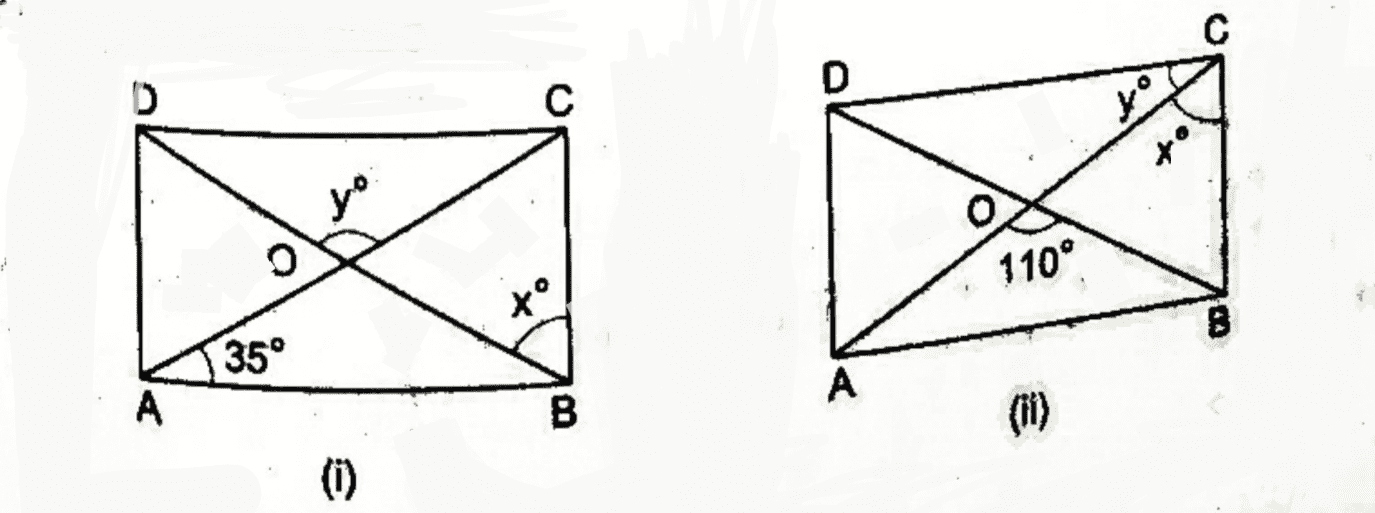Solution:

(i) ABCD is a rectangle

The diagonals of a rectangle are congruent and bisect each other. Therefore, in Δ$\Delta$ AOB, we have:

OA = OB

∠OAB = ∠OBA = 35°

x = 90° – 35° = 55° and ∠AOB = 180° – (35° + 35°) = 110°

y = ∠AOB = 110° [Vertically opposite angles]

Hence, x = 55° and y = 110°

(ii) In Δ$\Delta$ AOB, we have:

OA = OB

Now, ∠OAB = ∠OBA = 12 x 180° – 110° = 35°

y = ∠BAC = 35° [Interior alternate angles]

Also, x = 90° – y

x = 90° – 35° = 55°

Hence, x = 55° and y = 35°

Q.13: In the adjoining figure, ABCD is a square. S line segment CX cuts AB at X and the diagonal BD at O such that ∠COD =80° and ∠OXA = x°. Find the value of x°.

Sol:

The angles of a square are bisected by the diagonals.

∠OBX = 45° [Therefore, LABC = 90° and BD bisects LABC]

And ∠BOX= ∠COD = 80° [Vertically opposite angles]

In Δ$\Delta$BOX, we have:

∠AXO = ∠OBX+ ∠BOX [Exterior angle of Δ$\Delta$BOX]

= 45° + 80° = 125°

Therefore, x =125°

Q.14: In the adjoining figure, AL and CM are perpendiculars to the diagonal BD of a parallelogram ABCD. Prove that,

(i) Δ$\Delta$ALD = Δ$\Delta$CMB

(ii) AL = CM

Solution:

ABCD is a parallelogram.

(i) In Δ$\Delta$ ALD and Δ$\Delta$ CMB, we have:

Δ$\Delta$ ALD = Δ$\Delta$ CMB

(ii) As Δ$\Delta$ ALD = Δ$\Delta$ CMB

Therefore, AL = CM

Q.15: In the adjoining figure, ABCD is a parallelogram in which the bisectors of ∠A and ∠B intersect at a point P. Prove that ∠APB = 90°.

Sol:

ABCD is a parallelogram and ∠A and LB are adjacent angles.

∠A + ∠B= 180°.

∠A2 + ∠B2 = 1802 = 90°

In Δ$\Delta$APB, we have:

∠PAB = ∠A/2 and ∠PBA = ∠B/2

∠APB = 180 – (∠PAB + ∠PBA) [Angle sum property of triangle]

∠APB = 180 – ∠A2 + ∠B2

∠APB = 180 – 90 = 90° (Hence proved)

Q.16: In the adjoining figure, ABCD is a parallelogram. If P and Q are points on AD and BC respectively such that AP = 13AD$\frac{1}{3}AD$ and CQ =13AD$\frac{1}{3}AD$BC, prove that AQCP is a parallelogram.

Sol:

We have:

∠B= ∠D

AD= BC and AB = DC

Also, AD || BC and AB || DC

It is given that AP = 13AD and CQ = 13BC

AP = CQ

In Δ$\Delta$DPC and Δ$\Delta$BQA, we have:

AB =CD, ∠B= ∠D and DP =QB

i.e., Δ$\Delta$DPC = Δ$\Delta$BQA

PC = QA

Thus, in quadrilateral AQCP, we have:

AP = CQ . . . . . . . (i)

PC =QA . . . . . . . (ii)

Therefore, AQCP is a parallelogram

Q.17: In the adjoining figure, ABCD is a parallelogram whose diagonals intersect each other at O. A line segment EOF is drawn to meet AB at E and DC at F. Prove that OE = OF.

Sol:

In Δ$\Delta$ODF and Δ$\Delta$OBE, we have:

OD = 0B, ∠DOF = ∠BOE and ∠FDO = ∠OBE

i.e., Δ$\Delta$ODF = Δ$\Delta$OBE

OF = OE (Hence Proved)

Q.18: In the adjoining figure, ABCD is a parallelogram in which AB is produced to E so that BE = AB. Prove that ED bisects BC.

Sol:

In Δ$\Delta$ODC and Δ$\Delta$OEB. We have:

DC = BE, ∠COD = ∠BOE and ∠OCD = ∠OBE

i.e. Δ$\Delta$ODC = Δ$\Delta$OEB i.e. OC = 0B

We know that, BC = OC + 0B.

Therefore, ED bisects BC.

Q.19: In the adjoining figure, ABCD is a parallelogram and E is the midpoint of side BC. If DE and AB when produced meet at F, Prove that AF = 2 AB.

Sol:

Given: ABCD is a parallelogram.

BE = CE (E is the midpoint of BC)

DE and AB when produced meet at F

To prove: AF = 2AB

Proof:

In parallelogram ABCD, we have:

AB || DC

∠DCE = ∠EBF (Alternate interior angles)

In ADCE and ABFE, we have:

∠DCE = ∠EBF    (Proved above)

∠DEC = ∠BEF    (Vertically opposite angles)

Also, BE = CE (Given)

Δ$\Delta$DCE = Δ$\Delta$BFE (By ASA congruence rule)

DC = BF (CPCT)

But DC = AB, as ABCD is a parallelogram

DC = AB = BF . . . . . . . (i)

Now, AF = AB + BF . . . . . . . (ii)

From (i), we get:

AF = AB + AB = 2AB (Hence, proved)

Q.20: A Δ$\Delta$ABC is given. If lines are drawn through A, B, C parallel respectively to the sides BC, CA and AB, forming Δ$\Delta$PQR, as shown in the adjoining figure, show that BC = 12QR$\frac{1}{2}QR$.

Sol:

BC || QA and CA || QB

i.e., BCQA is a parallelogram.

BC = QA . . . . . . (i)

Similarly, BC || AR and AB || CR

i.e., BCRA is a parallelogram.

BC = AR . . . . . . . . . (ii)

But QR = QA + AR

From (i) and (ii), we get:

QR = BC + BC, QR = 2BC and BC = 12QR

Q.21: In the adjoining figure, Δ$\Delta$ABC is a triangle and through A, B, C lines are drawn, parallel respectively to BC, CA and AB, intersecting at P,Q and R. Prove that the perimeter of  Δ$\Delta$PQR is double the perimeter of  Δ$\Delta$ABC.

Sol:

Perimeter of Δ$\Delta$ABC = AB + BC + CA . . . . . . (i)

Perimeter of Δ$\Delta$PQR = PQ + QR + PR . . . . . . (ii)

BC || QA and CA || QB

i.e., BCQA is a parallelogram.

BC = QA . . . . . . (iii)

Similarly, BC || AR and AB || CR

i.e., BCRA is a parallelogram.

BC =AR . . . . . . (iv)

But, QR = QA + AR

From (iii) and (iv), we get:

QR = BC + BC

QR = 2BC

BC = 12QR

Similarly, CA = 12PQ and AB = 12PR

From (i) and (ii), we have:

Perimeter of Δ$\Delta$ABC = 12QR + 12PQ + 12PR = 12PR + QR + PQ

i.e., Perimeter of Δ$\Delta$ABC = 12(Perimeter of APQR)

Perimeter of Δ$\Delta$PQR = 2 Perimeter of Δ$\Delta$ABC.

Practise This Question

Who was the first scientist to define an element?